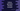# What is generator function in Python and how to use it## What is generator function in Python and how to use it:

A normal function returns a single value. You can call a function and it will return a number or a string etc. The generator function works in a different way. These types of functions returns a sequence of values. It doesn’t return only one value. It generates different values.

### Example of generator function:

Let’s take a look at the below program:

``````def hello():
yield 'Hello World !!'
yield 'Hello Universe !!'
yield 'Hello All !!'

hello_generator = hello()

print(next(hello_generator))
print(next(hello_generator))
print(next(hello_generator))``````
• Here, we are using yield instead of return. Each time the hello method is called, it returns different values. For the first time, it returns the first string, for the second call it returns the second string etc.
• If you call the function, it returns an iterator. So, the three print statements with next() will execute the first, second and the third yield.

It will print the below output:

``````Hello World !!
Hello Universe !!
Hello All !!``````

### Generator function with internal state:

If we use yield, it will maintain the internal state. For example, if we are using a variable with yield, and if the value of the variable got updated before the subsequent yield statements, it will maintain the state of that variable.

Let’s take a look at the below program:

``````def hello():
i = 10
yield 10

i += 10
yield i

i += 10
yield i

hello_generator = hello()

print(next(hello_generator))
print(next(hello_generator))
print(next(hello_generator))``````

In this example, we have initialized one variable i as 10. The yield statements are returning the i variable. Before each yield, its value is updated. It adds 10 to i. If you run this program, it will print the below output:

``````10
20
30``````

You can see that the state of i is maintained.

### Example of generator function to create a Fibonacci series function:

Let’s take an example of generator function to create a Fibonacci series function. This function will take a value of n as its parameter and yield first n Fibonacci series values.

``````def get_fibonacci(n):
first = 0
second = 1

for _ in range(n):
yield first
first, second = second, first + second

series = get_fibonacci(10)

for item in series:
print(item)``````

The get_fibonacci is a generator function that generates first n Fibonacci numbers. It takes the value of n as the parameter and yields the numbers.

If you run the above program, it will print the below output:

``````0
1
1
2
3
5
8
13
21
34``````

### Generator function yields the values only once:

Generator functions yields the values only once and if you use next after all items are generated, it will throw an error.

``````def my_generator():
yield "Hello !!"
yield "World !!"

g = my_generator()
print(next(g))
print(next(g))
print(next(g))``````

It will throw StopIteration exception:

``````Hello !!
World !!
Traceback (most recent call last):
File "example.py", line 9, in <module>
print(next(g))
StopIteration``````

### Generator expressions:

The generator expressions are anonymous generator functions. We can use generator expressions with simple generator functions. For example,

``````v = (i * 2 for i in range(5))

for item in v:
print(item)``````

It will print:

``````0
2
4
6
8``````

It is similar to list comprehension but it is more memory efficient as generator expression generates one item at a time instead of creating a full list. Also, it produces item only if it is asked. If we have thousands of values, a generator function will generate a value only if it is asked.